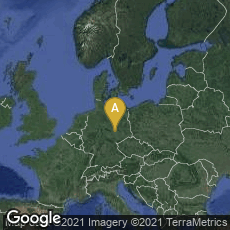A: Mitte, Halle (Saale), Sachsen-Anhalt, Germany

# Friedrich Ludwig Frege Founds Modern Mathematical Logic

1879

“. . . although a mere booklet of eighty-eight pages, it is perhaps the most important single work ever written in logic. Its fundamental contributions, among lesser points, are the truth-functional propositional calculus, the analysis of the proposition into function and argument(s) instead of subject and predicate, the theory of quantification, a system of logic in which derivations are carried out exclusively according to the form of the expressions, and a logical definition of the notion of mathematical sequence. Any single one of these achievements would suffice to secure the book a permanent place in the logician’s library” (Van Heijenoort, From Frege to Gödel (1967) 1).

“In his attempt to give a satisfactory definition of number and a rigorous foundation to arithmetic, Frege found ordinary language insufficient. To overcome the difficulties involved, he devised his Begriffschrift as a tool for analyzing and representing mathematical proofs completely and adequately. This tool has gradually developed into modern mathematical logic, of which Frege may justly be considered the creator“ (Dictionary of Scientific Biography article on Frege).